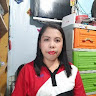## 7th# Sets## 10 questions

See Preview
• Multiple Choice
10 seconds
1 pt

List all the elements in A= {letters in the word "BOOK"}.

A= {B,O,K}

A= {B,O,O,K}

A= {B,K}• Multiple Choice
30 seconds
1 pt

Which symbol completes the statement correctly? {6}____{1,3,6}

$\epsilon$

$\subset$

$=$

$\not\subset$

• Multiple Choice
30 seconds
1 pt

Complete the statement correctly: Cow____ the set of all farm animals.

$\epsilon$

$\subset$

$\subseteq$

$\not\subset$